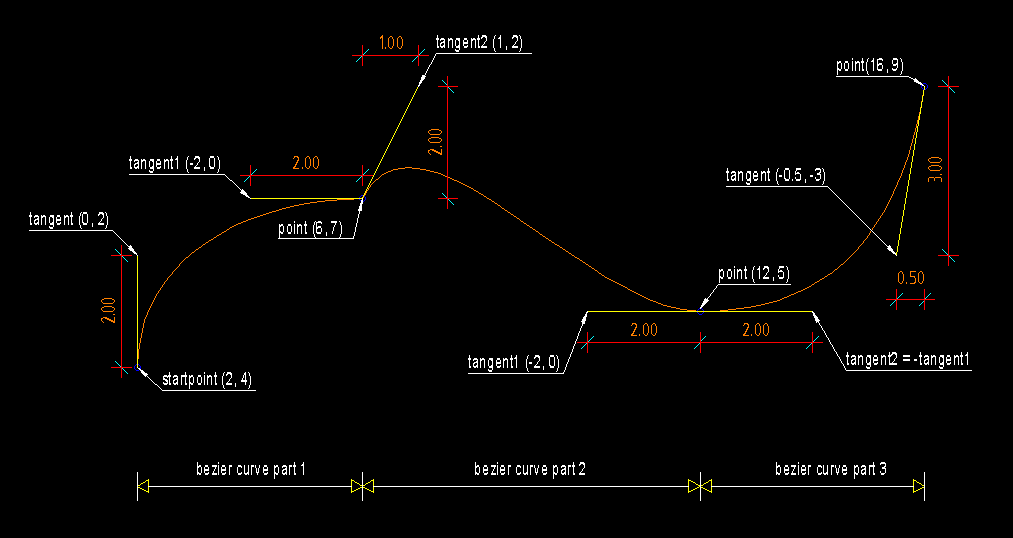# Bezier¶

Type: Composite Entity

Bezier curves are approximated by POLYLINE.

For an explanation of bezier curves see Wikipedia:

http://en.wikipedia.org/wiki/B%C3%A9zier_curve

`bezier`(color=const.BYLAYER, layer='0', linetype=None)
Parameters: color (int) – in range [1..255], 0 = BYBLOCK, 256 = BYLAYER layer (str) – layer name linetype (str) – linetype name, if not defined = BYLAYER

## Methods¶

`Bezier.start(point, tangent):`

Set start point and start tangent.

Parameters: point – 2D start point tangent – start tangent as 2D vector, example: (5, 0) means a horizontal tangent with a length of 5 drawing units
`Bezier.``append`(point, tangent1, tangent2=None, segments=20)

Append a control point with two control tangents.

Parameters: point – the control point as 2D point tangent1 – first control tangent as 2D vector left of point tangent2 – second control tangent as 2D vector right of point, if omitted tangent2 = -tangent1 segments (int) – count of line segments for polyline approximation, count of line segments from previous control point to this point.

## Example¶

```import dxfwrite
from dxfwrite import DXFEngine as dxf

def draw_control_point(point, tangent1, tangent2=(0, 0)):

name = 'bezier.dxf'
dwg = dxf.drawing(name)
bezier = dxf.bezier(color=4)

# define start point
bezier.start((2, 4), tangent=(0, 2))
draw_control_point((2, 4), (0, 2))

# append first point
bezier.append((6, 7), tangent1=(-2, 0), tangent2=(1, 2))
draw_control_point((6, 7), (-2, 0), (1, 2))

# tangent2 = -tangent1 = (+2, 0)
bezier.append((12, 5), tangent1=(-2, 0))
draw_control_point((12, 5), (-2, 0), (2, 0))

# for last point tangent2 is meaningless
bezier.append((16, 9), tangent1=(-0.5, -3))
draw_control_point((16, 9), (-0.5, -3))
```